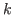Next: Introduction

# k = 1, 2 and Grundy numbers

Shunsuke Nakamura, Ryo Hanafusa, Wataru Ogasa, Takeru Kitagawa and Ryohei Miyadera

Kwansei Gakuin High School

runners@kwansei.ac.jp

### Abstract:

We study chocolate games that are variants of a game of Nim. We can cut the chocolate games in 3 directions, and we represent the chocolates with coordinates, whereare the maximum times you can cut them in each direction.
The coordinatesof the chocolates satisfy the inequalitiesfor.
Forwe prove a theorem for the L-state (loser's state), and the proof of this theorem can be easily generalized to the case of an arbitrary even number.
Forwe prove a theorem for the L-state (loser's state), and we need the theory of Grundy numbers to prove the theorem. The generalization of the case ofto the case of an arbitrary odd number is an open problem. The authors present beautiful graphs made by Grundy numbers of these chocolate games.